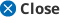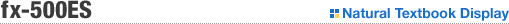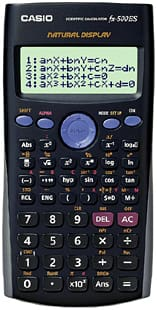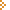Close upOne AAA-size(R03) battery 253 Functions / Full Dot Matrix Natural Textbook Display / Plastic Keys (Natural Input / Natural output)Equation calculation• Fraction calculation • Combination and Permutation • Logarithm log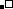• List-based STAT data editor • Standard deviation • Paired-variable statistics regression analysis • Table function • 7 variable memories • Comes with new slide on hard case